welefen## 通过开发 Babel 插件理解抽象语法树（AST）

Babel 是我们知道的将 ES6 代码转译为 ES5 代码且能安全稳定运行最好的工具，同时它允许开发者开发插件，能够在编译时期转换 JavaScript 的结构。

## 语言概述

``````var foo = { a: 1 };
var baz = foo.a = 2;
foo.a === 1;
baz.a === 2;

``````

``````var foo = mori.hashMap('a', 1);
var baz = mori.assoc(foo, 'a', 2);
mori.get(foo, 'a') === 1;
mori.get(baz, 'a') === 2;

``````

## Babel 概述### 解析

Babylon 是一个解析器，它可以将 JavaScript 字符串转换为对计算机来说更加友好的表现形式，称之为抽象语法树（AST）。

### 转换

babel-traverse 模块允许你浏览、分析和修改抽象语法树（AST）。

## 什么是抽象语法树（AST）

JavaScript 程序通常是由一系列的字符组成的，每一个在我们的大脑中都有一些可视的含义。这对我们来说非常方便，可以让我们使用匹配的字符（`[]`, `{}`, `()`），成对的字符（`''`, `""`）和缩进让程序解析起来更加简单。

``````var a = 3;
a + 5

``````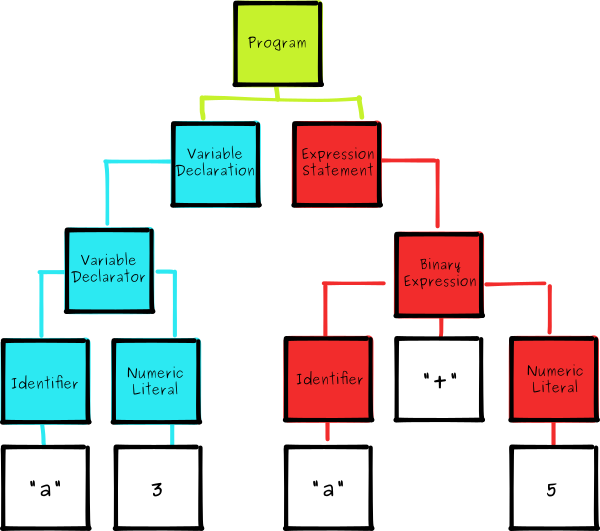1. 一个变量声明，将标识符 `a` 赋值为数值 `3`

2. 一个二元表达式语句，描述为标志符为 `a`，操作符 `+` 和数值 `5`

## 安装

``````mkdir moriscript && cd moriscript
npm init -y
npm install --save-dev babel-core

``````

``````// moriscript.js
module.exports = function(babel) {
var t = babel.types;
return {
visitor: {

}
};
};

``````

``````// run.js
var fs = require('fs');
var babel = require('babel-core');
var moriscript = require('./moriscript');

// read the filename from the command line arguments
var fileName = process.argv;

// read the code from this file
if(err) throw err;

// convert from a buffer to a string
var src = data.toString();

// use our plugin to transform the source
var out = babel.transform(src, {
plugins: [moriscript]
});

// print the generated code to screen
console.log(out.code);
});

``````

## 数组

MoriScript 首要和最重要的目标就是将普通对象和数组字面量转换为 Mori 对应的部分：HashMaps and Vectors。我们先来看看数组，它会相对简单一些：

``````var bar = [1, 2, 3];
// should become
var bar = mori.vector(1, 2, 3);

``````

``````{
"type": "ArrayExpression",
"elements": [
{
"type": "NumericLiteral",
"value": 1
},
{
"type": "NumericLiteral",
"value": 2
},
{
"type": "NumericLiteral",
"value": 3
}
]
}

``````

``````{
"type": "CallExpression",
"callee": {
"type": "MemberExpression",
"object": {
"type": "Identifier",
"name": "mori"
},
"property": {
"type": "Identifier",
"name": "vector"
}
},
"arguments": [
{
"type": "NumericLiteral",
"value": 1
},
{
"type": "NumericLiteral",
"value": 2
},
{
"type": "NumericLiteral",
"value": 3
}
]
}

``````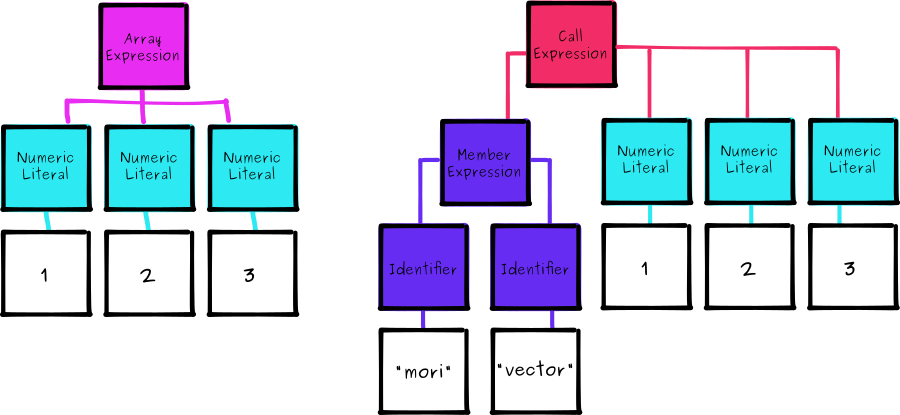``````module.exports = function(babel) {
var t = babel.types;
return {
visitor: {
ArrayExpression: function(path) {

}
}
};
};

``````

``````ArrayExpression: function(path) {
path.replaceWith(
t.callExpression(
t.memberExpression(t.identifier('mori'), t.identifier('vector')),
path.node.elements
)
);
}

``````

## 对象

``````var foo = { bar: 1 };
// should become
var foo = mori.hashMap('bar', 1);

``````

``````{
"type": "ObjectExpression",
"properties": [
{
"type": "ObjectProperty",
"key": {
"type": "Identifier",
"name": "bar"
},
"value": {
"type": "NumericLiteral",
"value": 1
}
}
]
}

``````

``````{
"type": "CallExpression",
"callee": {
"type": "MemberExpression",
"object": {
"type": "Identifier",
"name": "mori"
},
"property": {
"type": "Identifier",
"name": "hashMap"
}
},
"arguments": [
{
"type": "StringLiteral",
"value": "bar"
},
{
"type": "NumericLiteral",
"value": 1
}
]
}

``````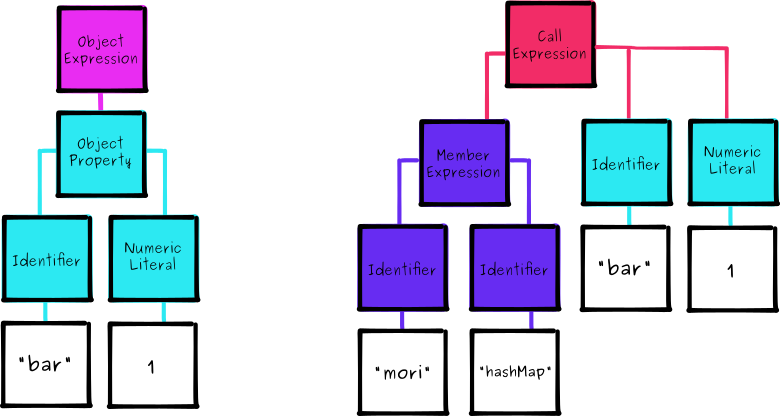``````ObjectExpression: function(path) {
var props = [];

path.node.properties.forEach(function(prop) {
props.push(
t.stringLiteral(prop.key.name),
prop.value.value
);
});

path.replaceWith(
t.callExpression(
t.memberExpression(t.identifier('mori'), t.identifier('hashMap')),
props
)
);
}

``````

``````// before
var foo = { bar: 1 };
// after
var foo = mori.hashMap(bar, 1);

``````

``````function moriMethod(name) {
return t.memberExpression(
t.identifier('mori'),
t.identifier(name)
);
}

// now rewrite
t.memberExpression(t.identifier('mori'), t.identifier('methodName'));
// as
moriMethod('methodName');

``````

``````mkdir test
echo -e "var foo = { a: 1 };\nvar baz = foo.a = 2;" > test/case.ms
node run.js test/case.ms

``````

``````var foo = mori.hashMap("a", 1);
var baz = foo.a = 2;

``````

## 赋值

``````foo.bar = 3;
// needs to become
mori.assoc(foo, 'bar', 3);

``````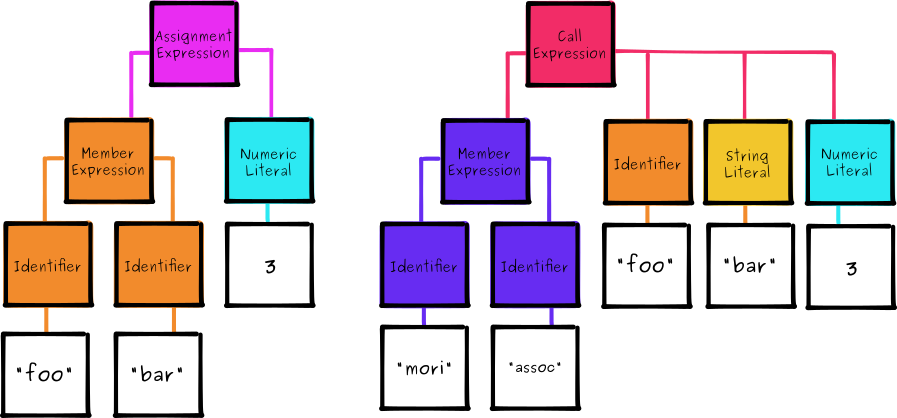``````AssignmentExpression: function(path) {
var lhs = path.node.left;
var rhs = path.node.right;

if(t.isMemberExpression(lhs)) {
if(t.isIdentifier(lhs.property)) {
lhs.property = t.stringLiteral(lhs.property.name);
}

path.replaceWith(
t.callExpression(
moriMethod('assoc'),
[lhs.object, lhs.property, rhs]
)
);
}
}

``````

`AssignmentExpressions` 处理方法先是初步检测表达式左侧是否有 `MemberExpression`（因为我们不希望类似 `var a = 3` 混淆进去），然后替换为 `CallExpression`，它通过 Mori `assoc` 方法创建的。

``````echo -e "foo.bar = 3;" >> test/case.ms
node run.js test/case.ms

\$ mori.assoc(foo, "bar", 3);

``````

## 成员

``````foo.bar;
// needs to become
mori.get(foo, 'bar');

``````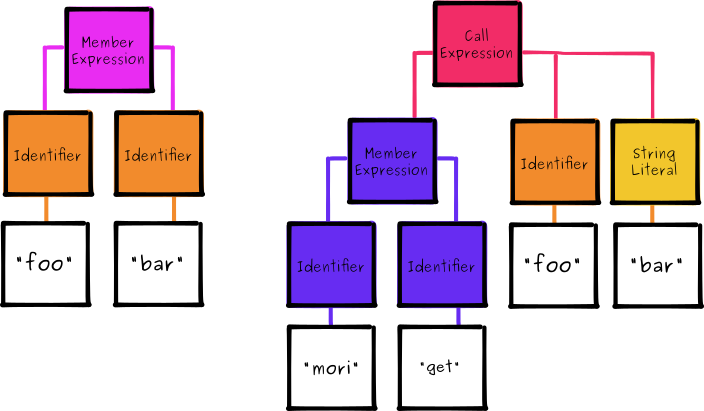``````MemberExpression: function(path) {
if(t.isAssignmentExpression(path.parent)) return;

if(t.isIdentifier(path.node.property)) {
path.node.property = t.stringLiteral(path.node.property.name);
}

path.replaceWith(
t.callExpression(
moriMethod('get'),
[path.node.object, path.node.property]
)
);
}

``````

``````function moriMethod(name) {
var expr = t.memberExpression(
t.identifier('mori'),
t.identifier(name)
);

expr.isClean = true;
return expr;
}

``````

``````MemberExpression: function(path) {
if(path.node.isClean) return;
if(t.isAssignmentExpression(path.parent)) return;

// ...
}

``````

``````echo -e "foo.bar" >> test/case.ms
node run.js test/case.ms

\$ mori.get(foo, "bar");

``````

## 结尾

``````2 | double | square

// would become

square(double(2))

``````

``````const doubleAndSquare = x => x | double | square

// would become

const doubleAndSquare = x => square(double(x));

// then use babel-preset-es2015

var doubleAndSquare = function doubleAndSquare(x) {
return square(double(x));
};

``````